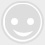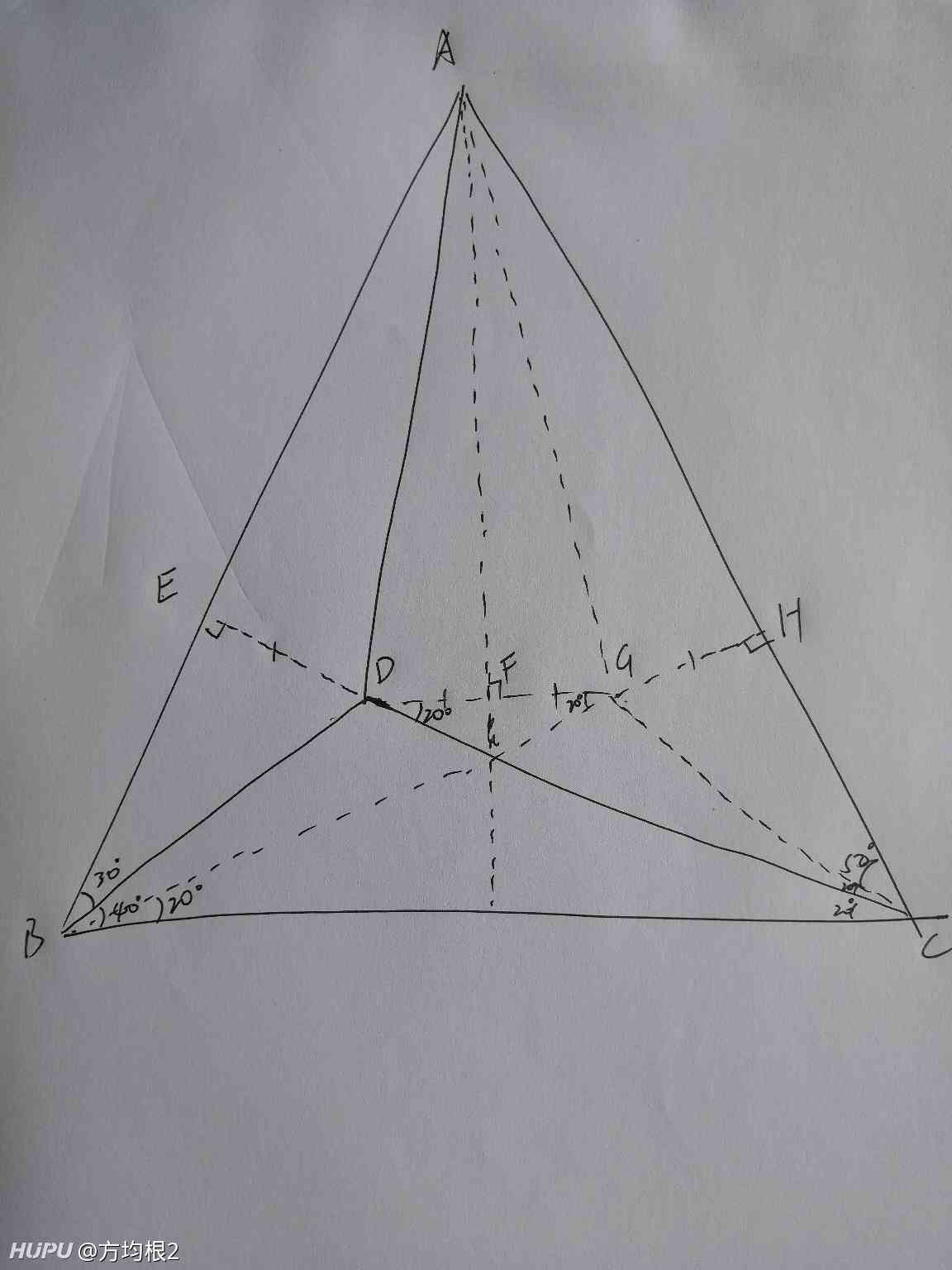# 我就不信这条Gai上有人能解的出来

### 最新回帖408 楼407 楼406 楼延长CD与AB交于E，作BH与AC交于H点，使∠HBC=20°，作CG与BH交于G点，连接DG，做AI⊥DG于F ∠ABC=∠ACB，△ABC为等腰三角形 易知∠CEB=90°，∠EAF=∠FAH=20° △ADG为等腰三角形，DF=FG △DGC为等腰三角形，DG=CG=2DF BD=2DE=CG=2DF, AE=AF,∠EAD=∠DAF=20°÷2=10° ∠DAH=30°404 楼

2016的不算太老，学校都是用2007402 楼30+40+20＝90，想到如果延长这条线就会出来直角了，然后根据外角和这个直角就会得出来问号是30度30度没错的，早上证明半天了，表达式应该有办法化简30度没错的，早上证明半天了，表达式应该有办法化简180-30-40-50-20=40，然后目测目标角占40的四分之三左右，然后蒙3004是真的🐮🍺，我现实中最低见过14的16版本有点旧啊

07最经典，虽然我也喜欢高版本的延长CD与AB交于E，作BH与AC交于H点，使∠HBC=20°，作CG与BH交于G点，连接DG，做AI⊥DG于F ∠ABC=∠ACB，△ABC为等腰三角形 易知∠CEB=90°，∠EAF=∠FAH=20° △ADG为等腰三角形，DF=FG △DGC为等腰三角形，DG=CG=2DF BD=2DE=CG=2DF, AE=AF,∠EAD=∠DAF=20°÷2=10° ∠DAH=30°延长CD与AB交于E，作BH与AC交于H点，使∠HBC=20°，作CG与BH交于G点，连接DG，做AI⊥DG于F ∠ABC=∠ACB，△ABC为等腰三角形 易知∠CEB=90°，∠EAF=∠FAH=20° △ADG为等腰三角形，DF=FG △DGC为等腰三角形，DG=CG=2DF BD=2DE=CG=2DF, AE=AF,∠EAD=∠DAF=20°÷2=10° ∠DAH=30°